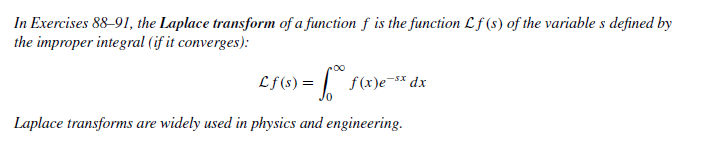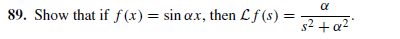# In Exercises 88-91, the Laplace transform of a function f is the function Lf (s) of the variable s defined by the improper integral (if it converges): Lf(s) = | f(x)e* dx Laplace transforms are widely used in physics and engineering. 89. Show that if f(x) = sin ax, then L f (s) = %3D s2 + a2"

Questionhelp_outlineImage TranscriptioncloseIn Exercises 88-91, the Laplace transform of a function f is the function Lf (s) of the variable s defined by the improper integral (if it converges): Lf(s) = | f(x)e* dx Laplace transforms are widely used in physics and engineering. fullscreenhelp_outlineImage Transcriptionclose89. Show that if f(x) = sin ax, then L f (s) = %3D s2 + a2" fullscreen

### Want to see this answer and more?

Experts are waiting 24/7 to provide step-by-step solutions in as fast as 30 minutes!*

*Response times may vary by subject and question complexity. Median response time is 34 minutes for paid subscribers and may be longer for promotional offers.
Tagged in
MathCalculus

### Other